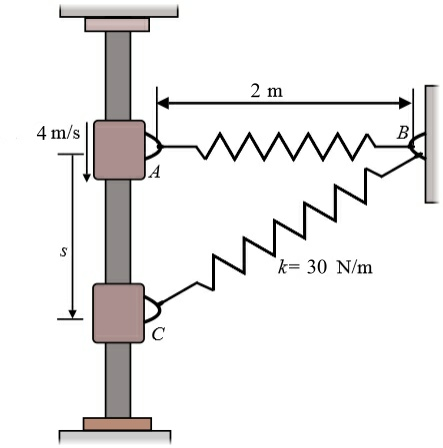# The 2 kg collar is given a downward velocity of 4 m/s when it is at A. (A) If the spring has an...

## Question:

The 2 kg collar is given a downward velocity of 4 m/s when it is at A.

(A) If the spring has an unstretched length of 1 m and stiffness of k=30 N/m, determine the velocity of the collar at s=1 m.## Energy.

The energy in an object is a transferable quantity that can be transferred from one to another. The energy can be represented as scalar quantity as it has magnitude, but the direction is not specified. It is measured in joules.

## Answer and Explanation:

Given data

• Mass of block is: {eq}m = 2\;{\rm{kg}} {/eq}.
• Initial velocity of block is: {eq}{v_A} = 4\;{{\rm{m}} {\left/ {\vphantom {{\rm{m}} {\rm{s}}}} \right. } {\rm{s}}} {/eq}.
• Unstreched length of spring is {eq}l = 1\;{\rm{m}} {/eq}.
• Spring stiffness is: {eq}k = 30\;{{\rm{N}} {\left/ {\vphantom {{\rm{N}} {\rm{m}}}} \right. } {\rm{m}}} {/eq}.
• Length AC is: {eq}s = 1\;{\rm{m}} {/eq}.
• Length AB is: {eq}t = 2\;{\rm{m}} {/eq}.

The length AB is taken as gravitational potential energy.

The formula for distance BC by Pythagoras theorem.

{eq}x = \left( {\sqrt {{t^2} + {s^2}} } \right) {/eq}

Substitute the given values in above formula.

{eq}\begin{align*} x &= \sqrt {{{\left( {2\;{\rm{m}}} \right)}^2} + {{\left( {1\;{\rm{m}}} \right)}^2}} \\ x &= 2.236\;{\rm{m}} \end{align*} {/eq}

The formula for velocity by conservation of energy.

{eq}\dfrac{1}{2}m{v_A}^2 + \dfrac{1}{2}k{\left( {t - l} \right)^2} = \dfrac{1}{2}m{v^2} - mgl + \dfrac{1}{2}k{\left( {x - l} \right)^2} {/eq}

Substitute the given values in above formula.

{eq}\begin{align*} \dfrac{1}{2} \times 2\;{\rm{kg}}{\left( {4\;{\rm{m/s}}} \right)^2} + \dfrac{1}{2} \times 30\;{\rm{N/m}}{\left( {2\;{\rm{m}} - 1\;{\rm{m}}} \right)^2} &= \dfrac{1}{2} \times 2\;{\rm{kg}} \times {v^2} - 2\;{\rm{kg}} \times {\rm{9}}{\rm{.81}}\;{\rm{m/}}{{\rm{s}}^2} \times 1\;{\rm{m}} + \dfrac{1}{2} \times 30\;{\rm{N/m}}{\left( {2.236\;{\rm{m}} - 1\;{\rm{m}}} \right)^2}\\ v &= 5.26\;{\rm{m/s}} \end{align*} {/eq}

Thus, the velocity is {eq}5.26\;{{\rm{m}} {\left/ {\vphantom {{\rm{m}} {\rm{s}}}} \right. } {\rm{s}}} {/eq}